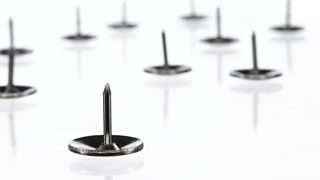Pressure on surfaces

You may have been warned about swinging around on one leg of a chair. Apart from the risk that you will damage the chair or hurt yourself, the chair leg can damage the floor. This is because it puts too much on the floor.

Calculating pressure

To calculate pressure, you need to know two things:

• the force or weight exerted
• the over which the force or weight is spread

Pressure is calculated using this equation:

pressure = force ÷ area

Example

A force of 20 N acts over an area of 4 m2. Calculate the pressure.

pressure = force ÷ area

= 20 N ÷ 4 m2 = 5 N/m2

Notice that the unit of pressure here is N/m2 (newtons per square metre). Sometimes you will see another unit being used. This is called the and it has the symbol Pa.

1 Pa = 1 N/m2, so in the example above the pressure is 5 Pa.

Using pressure

If you walk through snow, you usually sink into it. This is because your shoes have a small surface area. Your weight is only spread out over a small area, so the pressure on the snow is high. However, you will not sink so far into the snow if you are on skis. This is because your weight is spread out over a greater surface area, so the pressure on the snow is low.Drawing pins make good use of different pressures for the same force

Drawing pins have a large round end for your thumb to push. The round end has a large area, so it exerts a low pressure to your thumb. The sharp end has a very small area. The same pushing force produces a high pressure there, so it pushes into the notice board.

If you swing round on one leg of a chair, you put four times as much pressure on one point of the floor as you do if you sit properly. This is because four chair legs spread the pressure over four times more area than one chair leg can.